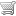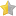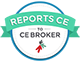No items in your cart.

# Mathematic Calculations and Applications for the Histology Laboratory (Online CE Course)(based on 53 customer ratings)

See all available courses »

### Continuing Education CreditsP.A.C.E.® Contact Hours (acceptable for AMT, ASCP, and state recertification): 1.5 hour(s)Course number 578-068-18, approved through 10/31/2020Florida Board of Clinical Laboratory Personnel Credit Hours - General (Histology): 1.5 hour(s)Course number 20-684156, approved through 9/1/2020

### Objectives

• Define terms associated with basic histology math processes.
• Apply unit conversions within the metric system, including the use of ratio and proportion.
• Covert temperatures between the Fahrenheit and Celsius systems.
• Calculate accurate concentrations of solutions, including molar concentrations, normal concentrations, percent concentrations, and conversions between percent and molar solutions.
• Perform dilution calculations to prepare working solutions from concentrated solutions.
• Determine the gravimetric factor in staining solution preparation and calculate the amount of dye needed in preparation of new stains.

### Customer Ratings(based on 53 customer ratings)

### Course Outline

Click on the links below to preview selected pages from this course.
• Introduction to Basic Laboratory Math
• Why do I Need to Understand the Math?
• Basic Math Applications in the Histology Laboratory
• The Metric System
• Making Temperature Conversions
• Conversion Between Fahrenheit and Celsius Scales
• Convert 50 degrees Celsius to degrees Fahrenheit.
• How many degrees Celsius is a temperature of -5 degrees Fahrenheit?
• Calculating Solution Concentrations
• Percentage Solutions
• What is a Percentage Solution?
• Calculating Percent Weight/Volume (% w/v)
• Calculating Percent Volume/Volume (% v/v)
• Calculating Solute Amounts for Percent Solutions
• In preparation for an ATPase stain to differentiate muscle fiber types, a histology student needs to prepare a 1% solution of calcium chloride (CaCl2)...
• A histotechnologist, in preparing alcian blue dye to stain for acid mucopolysaccharides, realizes there is little more than a gram of dye left in the ...
• 15 mL of ammonium hydroxide is diluted to 2.0 L with distilled water. What percentage solution would that be?
• Working Solutions
• Stock Solution Dilutions
• A histologist has 75% alcohol ready for use, but needs 30% alcohol for the procedure she wants to perform. How many mL of the 75% solution will she ne...
• Molar Solutions
• What is a Molar Solution?
• The histotechnician needs to prepare a 1M solution of sodium acetate (C2H3NaO2). A liter of solution is needed. How much sodium acetate is needed to p...
• The histotechnician in the previous question misread the concentration of sodium acetate she needs for her special stain. She needs to prepare only 50...
• How many grams of NaOH (GMW = 40) are needed to prepare 750 mL of a 0.5M solution ?
• Interchanging Between Percent Concentration and Molarity
• The histotechnician, needing a 2M solution of sulfuric acid (H2SO4), finds a 10% solution of H2SO4 on the shelf.Calculate the molarity of the 10% solu...
• Normality
• What is a Normal Solution?
• A histologist is prepping a stock solution of 2N KCl. How many grams of KCl will he need to make 500 mL of this solution?(K = 39.10 g; Cl = 35.45 g) V...
• How will a histotechnician prepare 300 mL of 0.4N H2SO4 for his special stains?
• How many grams of KOH must be weighed out to prepare 400 mL of a 0.5N solution? (K = 39.10; 0 = 16; H = 1)
• Interchanging of Molarity and Normality Concentrations
• Given a 2N solution of CaCl2, convert the solution expression to molarity. (valence of CaCl2 = 2)
• Determining the Gravimetric Factor for Stains
• What is the Gravimetric Factor?
• The histotechnician needs to prepare 150 mL of a 3% solution of aniline blue. She realizes the old stock dye is depleted and she will need to open a n...
• References